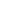### Prof.Tao Yu

Country/Region:China
University/Departmen:51 st Research Institute of CETC, Shanghai 201802, China
Brief introduction of research:

For nearly a decade, there has been a study of passive location technology. So far, more than 100 papers have been published.. A book is about to be published in Chinese technology by the national defense press.

The main results are:

1. Analytical analysis and potential application of multi-station differential equation

For planar multi-station positioning problem, if the auxiliary equations on the basis of the path difference measurement are constructed by using planar geometry, you can get a linear system. On the basis of linear solutions, two significant results are available:

(1) The simplified analysis for one-dimensional double-base direction finding can obtained a single medium point direction applicable to the long and short base line.

(2) The properties of the equivalent differential series between neighboring path difference can be obtained by the variable displacement for the one-dimensional double base direction finding.

2. Unambiguous phase difference positioning

There is periodicity ambiguity in phase difference measurement, so in the process of observation, The obtained observations themselves contain unknown differences of the   integer, and unknown parameters also vary with observations. Thus Directly from the mathematical equation, the phase difference location equation is not solvable.

The method of phase difference location is given indirectly by studying the unambiguous phase difference positioning based on the rate of change of phase difference. When the difference of integer of wavelengths is unknown, the phase difference rate can be obtained directly based on the phase difference measurement. This breakthrough research results have laid a very important foundation for engineering application of phase location.

3. Analytic method of bearing-only for kinematic parameters of target in fixed single station

Study on the analytical method for the target motion parameters only by means of azimuth measurement for fixed single station is more than 60 years of history. By introducing the equation of self time difference measurement and taking full advantage of the simple plane geometry relation, the analytic formula of bearing-only for single station passive location is given.

4. Two planes cooperative localization method without any baseline length using external radiation sources

A cooperative location method using two planes based on external radiation source is presented. Its most notable feature is that not only do not need to know the baseline length between the detection station and the radiation source, also do not need to know the baseline length between two planes. The existing methods must at least know the distance between the detection station and third party radiation source distance.

#### 相关阅读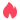热门推荐X•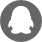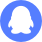QQ客服
•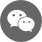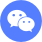微信客服

扫码添加廖老师•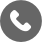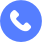客服电话

电话:020-28101017

•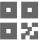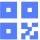公众号

关注AEIC公众号查看更多资讯•